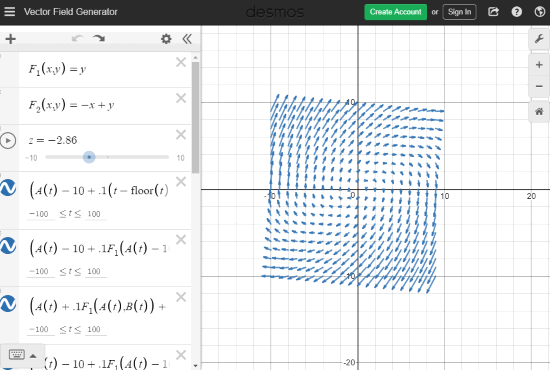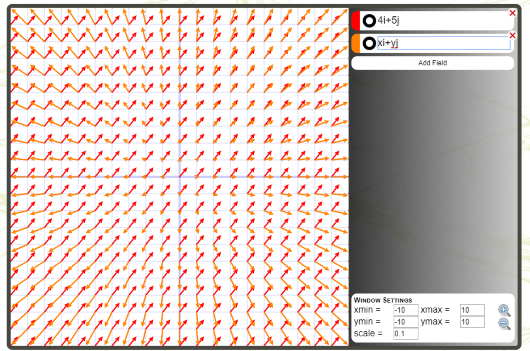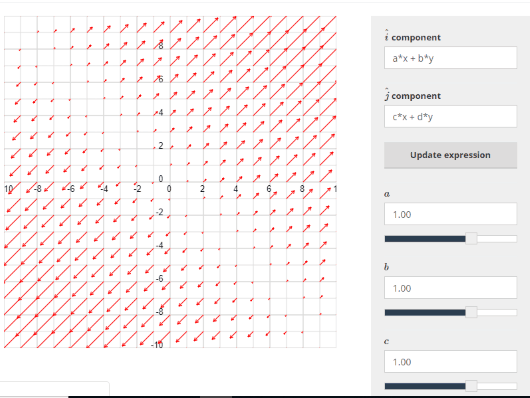Editor Ratings:
User Ratings:
[Total: 0 Average: 0]

Here are 3 free online vector field grapher websites. These websites help you view vector field in a plane or graph where arrows are shown for the magnitude and direction of the vector field. All of these websites are extremely easy to use. You don’t have to create some account or do some other efforts to plot vector field graph.

There will be a simple interface where you can add vector equations and values for the items present in the equation. After that, the output will be visible to you immediately on the website interface.

All these websites also come with the option to save the vector field graph to PC as PNG/JPG image. So, let’s check these free online vector field graphers one by one.

## Vector Field GeneratorVector Field Generator (provided by Desmos.com) is one of the best options here. Here is its homepage. On the left section, there are preset vector equations. You can use them as a sample or simply delete or edit any of those equations to create the vector field. As soon as you add some equation, you can see the output graph or vector field on the right side.

You can also create a free account but it doesn’t help you store multiple vector fields. So, it might not be that much help to create an account, but it is good if you want to save a vector field online and then edit it later. It also comes with other good features. For example, you can share the graph by generating a unique URL, zoom in and out vector field graph, etc.

## Vector Field Online GraphingVector Field Online Graphing (Homepage) is a very simple website where you can enter multiple vector equations and then vector fields are displayed with different arrows based on the input equations. For each equation, arrows are visible with different colors. This helps to identify which vector field belongs to which equation.

You can also adjust the graph options. That means you can set xmin, xmax, ymin, ymax, and scale values. All the changes added by you are reflected in the vector field. When the final output is ready, you can right-click on the graph and then save it as PNG image.

## Vector Field PlotterVector Filed Plotter (Homepage) is a very simple vector field grapher website. You can use ‘i’ and ‘j’ components, which help to add vector equations or expressions. In ‘i’ and ‘j’ fields or boxes, there will be a, b, c, and d items in the vector equations. For each of these items, a separate box is available to enter the values. So, use those boxes to enter values and update the equation.

When the equation is added, you can see the output vector field graph on the left part. To save the output vector field graph, you need to use the right-click menu. After that, you will be able to save the output in PNG format.

### The Conclusion:

Creating a vector field graph with these free websites is pretty simple. You just need to add vector equations and check the output. Among these free vector field grapher websites, the first website is the most useful. The reason is it brings a user-friendly interface where preset equations are already available with output preview. So, it becomes easier to plot some vector field graph.

 Editor Ratings: User Ratings:[Total: 0 Average: 0]